Successfully reported this slideshow.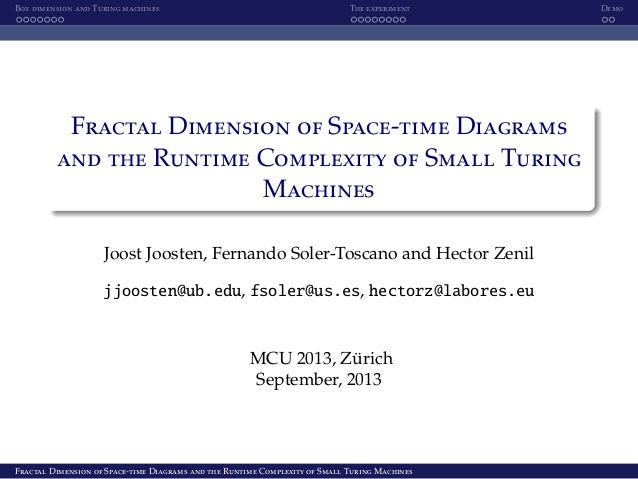Upcoming SlideShare
×

of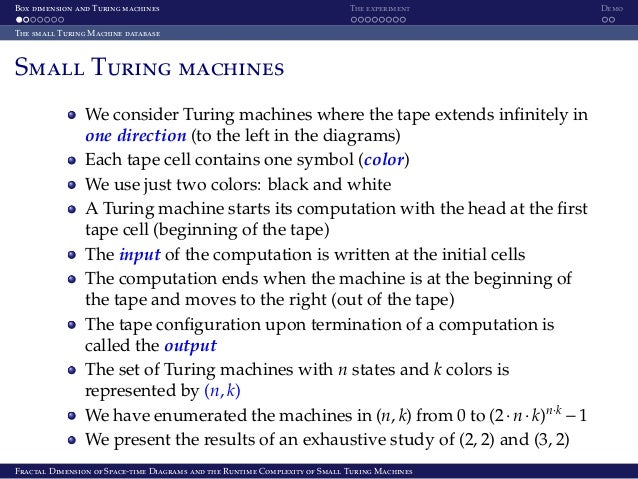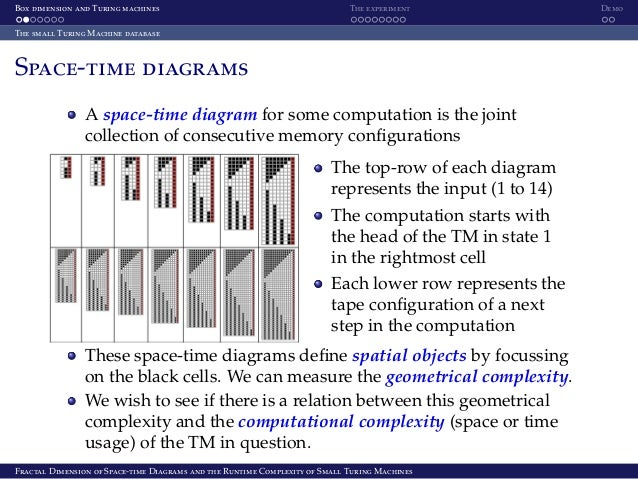4

Share

# Fractal Dimension of Space-time Diagrams and the Runtime Complexity of Small Turing Machines

Complexity measures are designed to capture complex behaviour and to quantify how complex that particular behaviour is. If a certain phenomenon is genuinely complex this means that it does not all of a sudden becomes simple by just translating the phenomenon to a different setting or framework with a different complexity value. It is in this sense that we expect different complexity measures from possibly entirely different fields to be related to each other. This work presents our work on a beautiful connection between the fractal dimension of space-time diagrams of Turing machines and their time complexity. Presented at Machines, Computations and Universality (MCU) 2013, Zurich, Switzerland.

See all

See all

### Fractal Dimension of Space-time Diagrams and the Runtime Complexity of Small Turing Machines

1. 1. Box dimension and Turing machines The experiment Demo Fractal Dimension of Space-time Diagrams and the Runtime Complexity of Small Turing Machines Joost Joosten, Fernando Soler-Toscano and Hector Zenil jjoosten@ub.edu, fsoler@us.es, hectorz@labores.eu MCU 2013, Z¨urich September, 2013 Fractal Dimension of Space-time Diagrams and the Runtime Complexity of Small Turing Machines
2. 2. Box dimension and Turing machines The experiment Demo The small Turing Machine database Small Turing machines We consider Turing machines where the tape extends inﬁnitely in one direction (to the left in the diagrams) Each tape cell contains one symbol (color) We use just two colors: black and white A Turing machine starts its computation with the head at the ﬁrst tape cell (beginning of the tape) The input of the computation is written at the initial cells The computation ends when the machine is at the beginning of the tape and moves to the right (out of the tape) The tape conﬁguration upon termination of a computation is called the output The set of Turing machines with n states and k colors is represented by (n, k) We have enumerated the machines in (n, k) from 0 to (2·n·k)n·k −1 We present the results of an exhaustive study of (2, 2) and (3, 2) Fractal Dimension of Space-time Diagrams and the Runtime Complexity of Small Turing Machines
3. 3. Box dimension and Turing machines The experiment Demo The small Turing Machine database Space-time diagrams A space-time diagram for some computation is the joint collection of consecutive memory conﬁgurations The top-row of each diagram represents the input (1 to 14) The computation starts with the head of the TM in state 1 in the rightmost cell Each lower row represents the tape conﬁguration of a next step in the computation These space-time diagrams deﬁne spatial objects by focussing on the black cells. We can measure the geometrical complexity. We wish to see if there is a relation between this geometrical complexity and the computational complexity (space or time usage) of the TM in question. Fractal Dimension of Space-time Diagrams and the Runtime Complexity of Small Turing Machines
4. 4. Box dimension and Turing machines The experiment Demo Fractal dimensions Box dimension The notion of Box dimension was introduced by Kolmogorov as a simpliﬁcation of the Hausdorﬀ dimension Suppose we have a mathematical object S of bounded size. The idea is to cover S with boxes in Rn and estimate the “volume” V(S) of S as function of the total number of boxes N(S) needed to cover S: V(S) = limr↓0 rd N(S, r) Definition (Box dimension) Let S be some spatial object that can be embedded in some Rn , let N(S, r) denote the minimal number of boxes of size r needed to fully cover S. The Box dimension of S is denoted by δ(S) and is deﬁned by δ(S) := lim r↓0 log(N(S, r)) log(1 r ) in case this limit is well deﬁned. In all other cases we shall say that δ(S) is undeﬁned. Fractal Dimension of Space-time Diagrams and the Runtime Complexity of Small Turing Machines
5. 5. Box dimension and Turing machines The experiment Demo Fractal dimensions Box dimension for Space-Time diagrams We adapt the notion of Box dimension to space-time diagrams Clearly, for each input on which the TM halts the corresponding space-time diagram has dimension 2: it’s a piece of surface It gets interesting when we consider limiting behavior of the TM Definition (Box dimension of a Turing machine) Let τ be a TM that converges on inﬁnitely many input values x. In case τ(x) ↓, let N(τ, x) denote the number of black cells in the space-time diagram of τ on input x and let t(τ, x) denote the number of steps needed for τ to halt on x. We will deﬁne the Box dimension of a TM τ and denote it by d(τ). In case t(τ, x) is constant from some x onwards, we deﬁne d(τ) := 2. Otherwise, we deﬁne d(τ) := lim inf x→∞ log N(τ, x) log t(τ, x) . Fractal Dimension of Space-time Diagrams and the Runtime Complexity of Small Turing Machines
6. 6. Box dimension and Turing machines The experiment Demo The Space-Time Theorem and applications The Space-time Theorem: upper and lower bounds Theorem (Space-time Theorem: upper bound) Let us, for a given TM τ, denote by s(x) the number of cells visited by τ on input x, and let t(x) denote the number of computation steps it took τ to terminate on input x. If lim infx→∞ log(s(x)) log(t(x)) = n then d(τ) ≤ 1 + n. Lemma (lower bound) For each TM τ we have that d(τ) ≥ 1. Fractal Dimension of Space-time Diagrams and the Runtime Complexity of Small Turing Machines
7. 7. Box dimension and Turing machines The experiment Demo The Space-Time Theorem and applications The Upper Bound Conjecture Lemma In case a TM τ uses polynomial space, and runs super-polynomial time we have that d(τ) = 1. More in general, if τ uses space sτ(x) and time tτ(x) on input x then lim inf x→∞ log sτ(x) log tτ(x) = 0 ⇐⇒ d(τ) = 1. Conjecture (Upper Bound Conjecture) We conjecture that for each n ∈ ω and each TM τ in (n, 2) space that d(τ) = 1 + lim inf x→∞ log sτ(x) log tτ(x) Fractal Dimension of Space-time Diagrams and the Runtime Complexity of Small Turing Machines
8. 8. Box dimension and Turing machines The experiment Demo The Space-Time Theorem and applications The Space-Time Theorem and P versus NP Using the previous Lemma, we can state a separation of P and NP in terms of dimensions: Let Π be some NP-complete problem If for each PSPACE Turing machine τ that decides Π we have that d(τ) = 1, then P NP. Clearly, this does not constitute a real strategy since, in general, it is undecidable whether d(τ) = 1 Fractal Dimension of Space-time Diagrams and the Runtime Complexity of Small Turing Machines
9. 9. Box dimension and Turing machines The experiment Demo Methodology Slow convergence Our aim is to use computer experiments to compute the Box dimension of all TMs τ where d(τ) is not predicted by any theoretical result. A substantial complication is caused by the occurrence of logarithms in d(τ) As a consequence, increase in precision of d(τ) requires exponentially larger inputs For (2, 2) TM 346 we know that its Box dimension is 2, but we can see in the picture how slow the rate of convergence is Our way out here is to apply numerical and mathematical analysis to the functions involved so that we can retrieve their limit behavior. We are interested in three diﬀerent functions: tτ(x), number of time-steps needed for τ to halt on input x Nτ(x), number of black cells in the space-time diagram of τ on input x sτ(x), number of tape cells visited by τ on input x Fractal Dimension of Space-time Diagrams and the Runtime Complexity of Small Turing Machines
10. 10. Box dimension and Turing machines The experiment Demo Methodology Steps followed Each TM in (2, 2) also occurs in (3, 2) so for the ﬁnal results it suﬃces to focus on this data-set. We isolated the TMs for which there is no theorem that predicts the corresponding dimension. Boxes Runtime Space Machines O(n3) O(n2) O(n) 3358 O(n4) O(n3) O(n) 6 o(P) o(P) o(P) 14 Per TM τ, we determined its functions sτ(x) (space), tτ(x) (time) and Nτ(x) (black cells). We used FindSequenceFunction and other Mathematica functions. This is the hard work! Per TM τ, we computed its dimension d(τ) as d(τ) = lim infx→∞ log(Nτ(x)) log(tτ(x)) Per TM τ, we compared its dimension d(τ) to its theoretical upper bound 1 + lim infx→∞ log(sτ(x)) log(tτ(x)) Fractal Dimension of Space-time Diagrams and the Runtime Complexity of Small Turing Machines
11. 11. Box dimension and Turing machines The experiment Demo Methodology Alternating convergent behavior Some machines have alternating asymptotic behavior This is the most extreme example (TM 1,728,529): For convenience we have changed the orientation of the diagrams so that time ‘goes from left to right’ instead of from ‘top to bottom’. In a sense this TM incorporates two diﬀerent algorithms to compute this output: one in linear time, the other, in exponential time. We have found alternating sequences of periodicity 2, 3 and 6 The periodicity typically reﬂects either the number of states, the number of colors, or a divisor of their product. It is this alternating behavior which complicated the analysis of the data set in a straight-forward automated fashion. Fractal Dimension of Space-time Diagrams and the Runtime Complexity of Small Turing Machines
12. 12. Box dimension and Turing machines The experiment Demo Most salient results of the experiment Findings in (2, 2) space In (2, 2) there was a total of 74 diﬀerent functions. Only 5 of them where computed by some super-linear time TMs In total, in (2, 2) space, there are only 7 TMs that run in super-polynomial time. Three of them run in exp-time, all computing the tape-identity. The other four (see below) TMs compute diﬀerent functions (that roughly double the tape input) All these four TMs perform in quadratic time and linear space. The dimension for these functions is 3 2 . This is exactly the upper bound as predicted by the Space-Time Theorem. Fractal Dimension of Space-time Diagrams and the Runtime Complexity of Small Turing Machines
13. 13. Box dimension and Turing machines The experiment Demo Most salient results of the experiment Findings in (3, 2) space The (3, 2) space contains 2,985,984 many diﬀerent TMs which compute 3,886 diﬀerent functions Almost all TMs used at most linear space for their computations The only exception to this was when the TM used exponential space Busy Beaver: we call a TM β a Busy Beaver whenever for each TM τ, there is some value x0 so that for all x ≥ x0 we have tβ(x) ≥ tτ(x) Twin Machines 599,063 and 666,364 are the Busy Beavers in (3,2) space, running in exponential space and time. They compute the largest runtime, space and boxes sequences. They also produce the longest output strings. Fractal dim.: 3/2 Fractal Dimension of Space-time Diagrams and the Runtime Complexity of Small Turing Machines
14. 14. Box dimension and Turing machines The experiment Demo Most salient results of the experiment The space-time theorem revisited One of our most important empirical ﬁndings is that the upper bound as given by the Space-Time Theorem is actually always attained in (3, 2) space. Finding 1 For all TMs τ in (3,2) space we found that d(τ) = 1 + lim inf x→∞ log(sτ(x)) log(tτ(x)) as conjectured in the Upper Bound Conjecture that this holds in general for TMs with a larger number of states. Fractal Dimension of Space-time Diagrams and the Runtime Complexity of Small Turing Machines
15. 15. Box dimension and Turing machines The experiment Demo Most salient results of the experiment Other findings Finding 2 For all TMs τ in (3,2) space we found that d(τ) = 1 if and only if the TM ran in super-polynomial time using polynomial space. We suspect that this equivalence holds no longer true in higher spaces, i.e., spaces (n, 2) for n > 3. Finding 3 For all TMs τ in (3,2) space we found that d(τ) = 2 if and only if the TM ran in at most linear time. It is unknown if this equivalence holds true in higher spaces (the “if” part holds in general and is proven in previous lemmas) Fractal Dimension of Space-time Diagrams and the Runtime Complexity of Small Turing Machines
16. 16. Box dimension and Turing machines The experiment Demo Most salient results of the experiment Richness in the (3, 2) space We have found two symmetric performers for even inputs This can only occur in machines computing the tape identity and requires strong conditions It’s surprising that such constraints can be met in (3, 2) Fractal Dimension of Space-time Diagrams and the Runtime Complexity of Small Turing Machines
17. 17. Box dimension and Turing machines The experiment Demo Demo We have prepared a demo to visualize the space-time diagrams for several TMs in (3, 2) It will be published in Wolfram Demonstrations Project, and it’s available upon request Fractal Dimension of Space-time Diagrams and the Runtime Complexity of Small Turing Machines
18. 18. Box dimension and Turing machines The experiment Demo Demo Fractal Dimension of Space-time Diagrams and the Runtime Complexity of Small Turing Machines
•#### AnandSwami4

Jun. 13, 2020
•#### VikashKumar1344

May. 9, 2018
•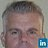#### MPerryPhDMBAMPHMSFAM

May. 16, 2017
•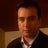Sep. 6, 2013

Total views

2,721

On Slideshare

0

From embeds

0

Number of embeds

2

22

Shares

0